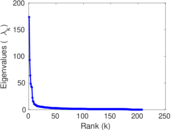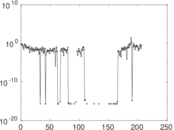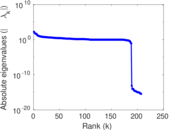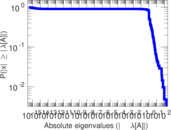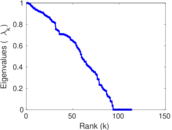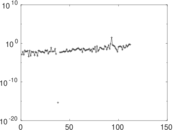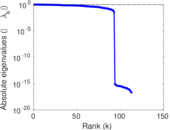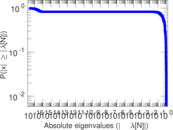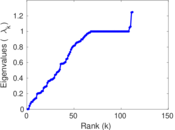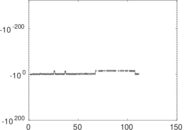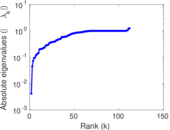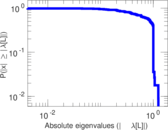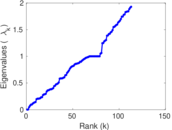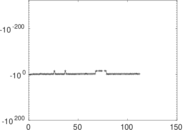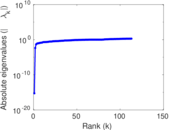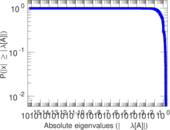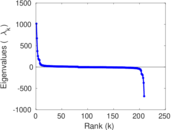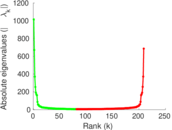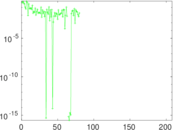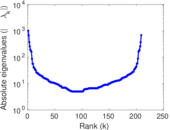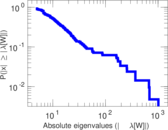# Wikibooks edits (la)

This is the bipartite edit network of the Latin Wikibooks. It contains users and pages from the Latin Wikibooks, connected by edit events. Each edge represents an edit. The dataset includes the timestamp of each edit.

 Code `bla` Internal name `edit-lawikibooks` Name Wikibooks edits (la) Data source http://dumps.wikimedia.org/ AvailabilityDataset is available for download Consistency checkDataset passed all tests Category Authorship network Dataset timestamp 2017-10-20 Node meaning User, article Edge meaning Edit Network formatBipartite, undirected Edge typeUnweighted, multiple edges Temporal dataEdges are annotated with timestamps

## Statistics

 Size n = 1,014 Left size n1 = 209 Right size n2 = 805 Volume m = 2,950 Unique edge count m̿ = 1,229 Wedge count s = 50,143 Claw count z = 2,584,797 Cross count x = 112,102,288 Square count q = 6,349 4-Tour count T4 = 254,786 Maximum degree dmax = 955 Maximum left degree d1max = 955 Maximum right degree d2max = 153 Average degree d = 5.818 54 Average left degree d1 = 14.114 8 Average right degree d2 = 3.664 60 Fill p = 0.007 304 82 Average edge multiplicity m̃ = 2.400 33 Size of LCC N = 740 Diameter δ = 13 50-Percentile effective diameter δ0.5 = 4.364 84 90-Percentile effective diameter δ0.9 = 9.163 20 Median distance δM = 5 Mean distance δm = 5.588 81 Gini coefficient G = 0.758 200 Balanced inequality ratio P = 0.194 915 Left balanced inequality ratio P1 = 0.131 186 Right balanced inequality ratio P2 = 0.263 051 Relative edge distribution entropy Her = 0.840 352 Power law exponent γ = 3.747 45 Tail power law exponent γt = 2.201 00 Tail power law exponent with p γ3 = 2.201 00 p-value p = 0.283 000 Left tail power law exponent with p γ3,1 = 1.891 00 Left p-value p1 = 0.367 000 Right tail power law exponent with p γ3,2 = 3.471 00 Right p-value p2 = 0.002 000 00 Degree assortativity ρ = −0.200 576 Degree assortativity p-value pρ = 1.277 76 × 10−12 Spectral norm α = 173.568 Algebraic connectivity a = 0.004 155 50 Spectral separation |λ1[A] / λ2[A]| = 1.865 30 Controllability C = 630 Relative controllability Cr = 0.626 243

## Plots

### Fruchterman–Reingold graph drawing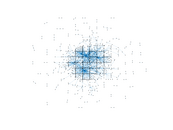### Degree distribution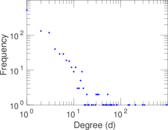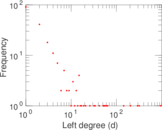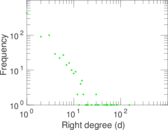### Cumulative degree distribution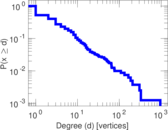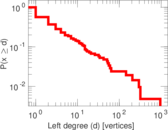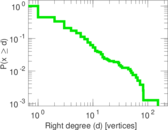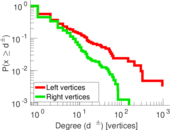### Lorenz curve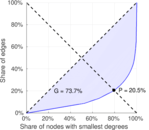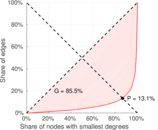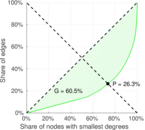### Spectral distribution of the adjacency matrix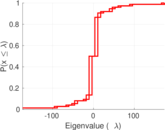### Spectral distribution of the normalized adjacency matrix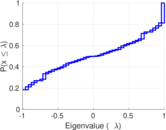### Spectral distribution of the Laplacian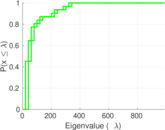### Spectral graph drawing based on the adjacency matrix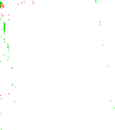### Spectral graph drawing based on the Laplacian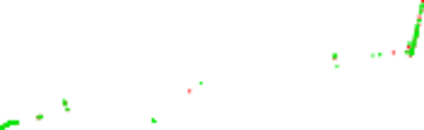### Spectral graph drawing based on the normalized adjacency matrix### Degree assortativity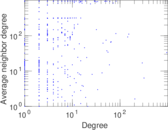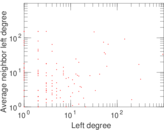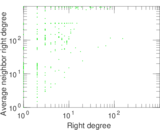### Zipf plot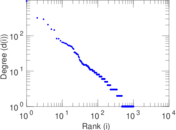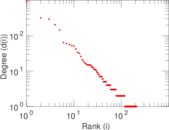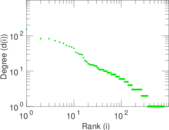### Hop distribution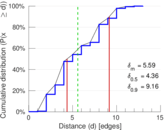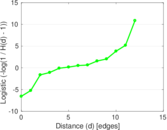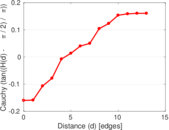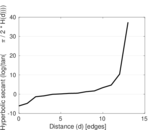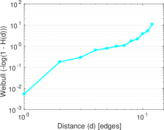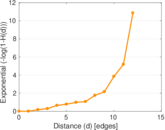### Double Laplacian graph drawing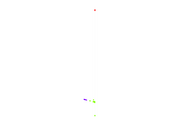### Delaunay graph drawing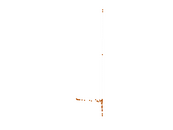### Edge weight/multiplicity distribution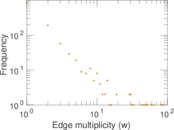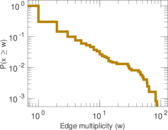### Temporal distribution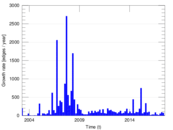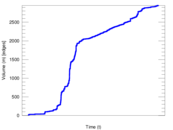### Temporal hop distribution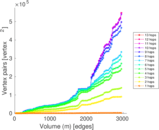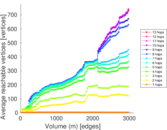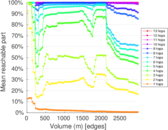### Diameter/density evolution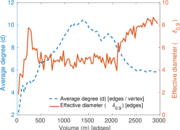### Matrix decompositions plots